# Problems of the Week

Contribute a problem

# 2018-02-12 Intermediate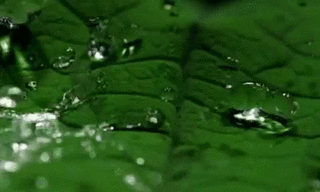The lotus flower is self-cleaning. Water slides off with ease, and even incredibly viscous substances like honey and glue will come right off.

Like other leaves, the lotus leaf has a waxy cuticle. However, the lotus leaf is significantly more hydrophobic.

Below are illustrations of leaf surfaces. Which surface is most likely to resemble that of a real lotus leaf?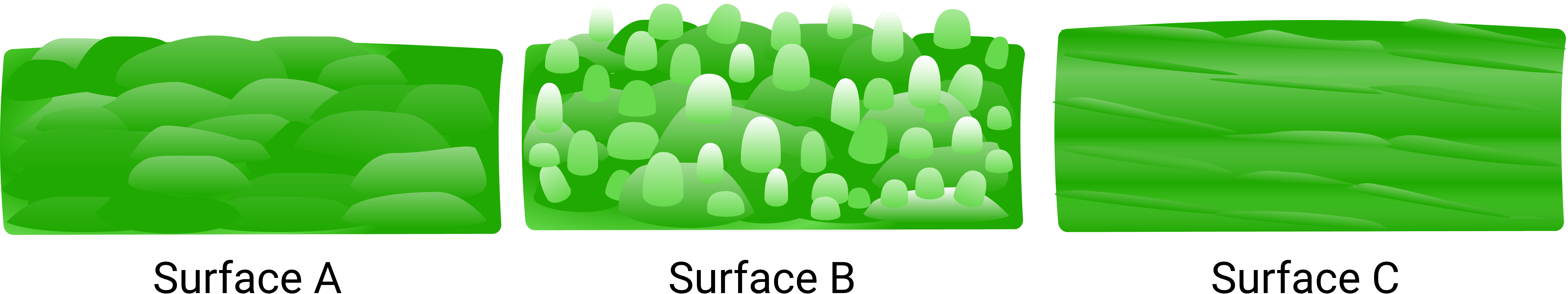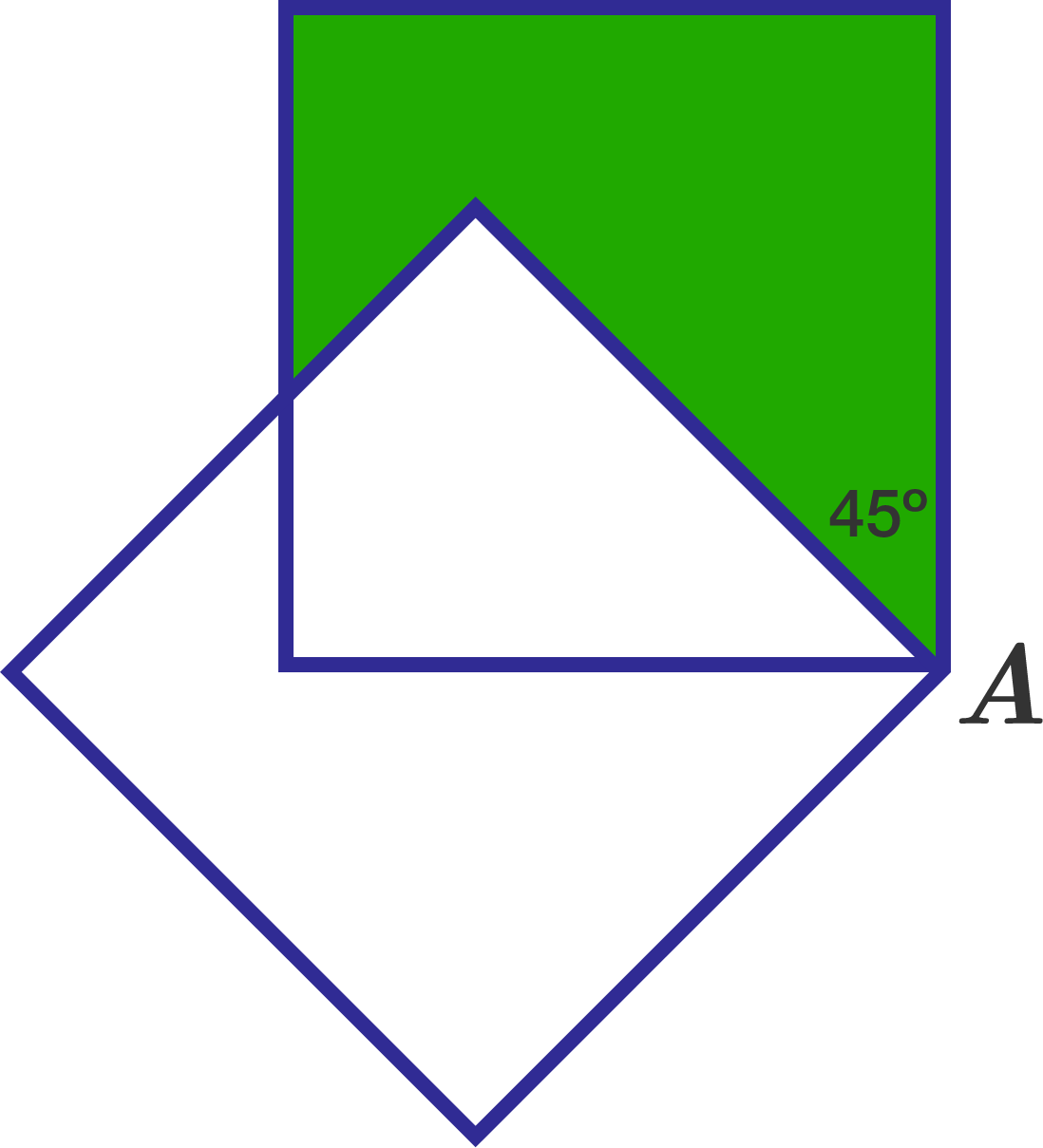A unit square is rotated $45^\circ$ counterclockwise about one of its vertices, $A.$

What is the green area?

Krishna and Satvik are playing a game called 3-Point Shattering, which has the following rules:

1. Krishna chooses where to place 3 points on a large sheet of paper.
2. Satvik marks each point either blue or red.
3. Krishna tries to draw a line such that all the blue points are on one side of it and all the red points are on the other.

Krishna wins if he can do Step 3. Otherwise, Satvik wins. Here are some examples of how Krishna can win: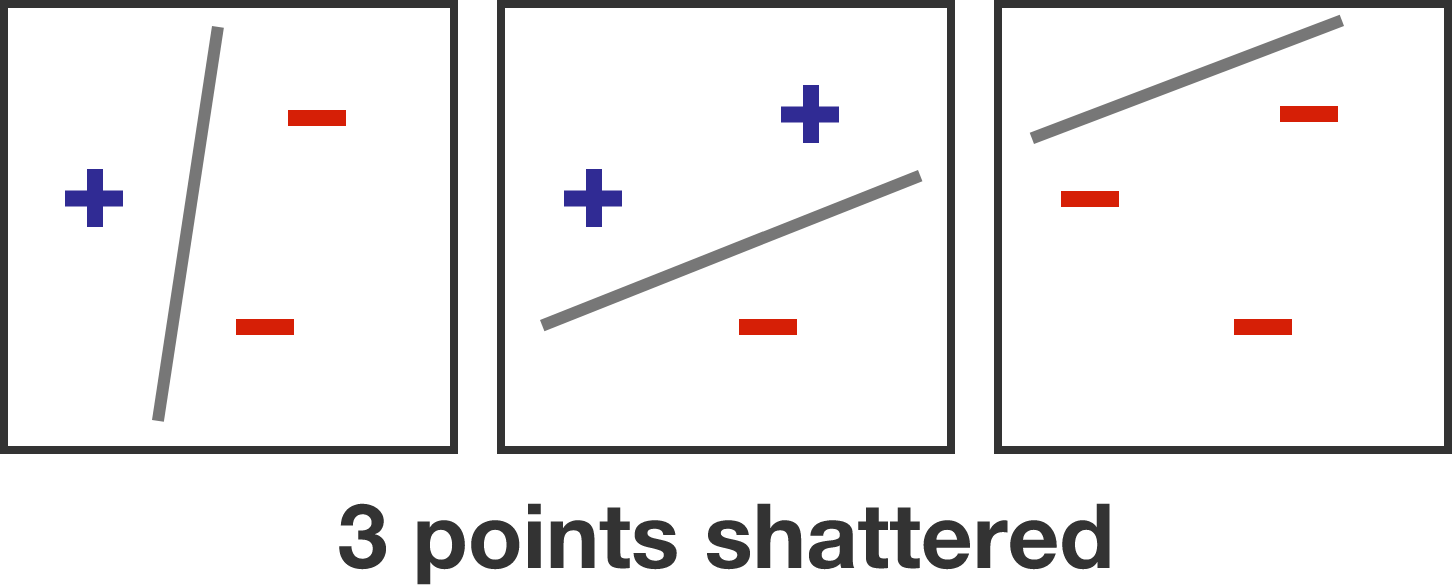Notice that Krishna can still win if Satvik marks all the points the same color.

However, if they played a slightly different game, 4-Point Shattering, where Krishna starts by placing 4 points, who has a winning strategy?

Below is a multiplication table up to $y \times y.$ Find $y$ such that the sum of all the products in the blue square equals 90000.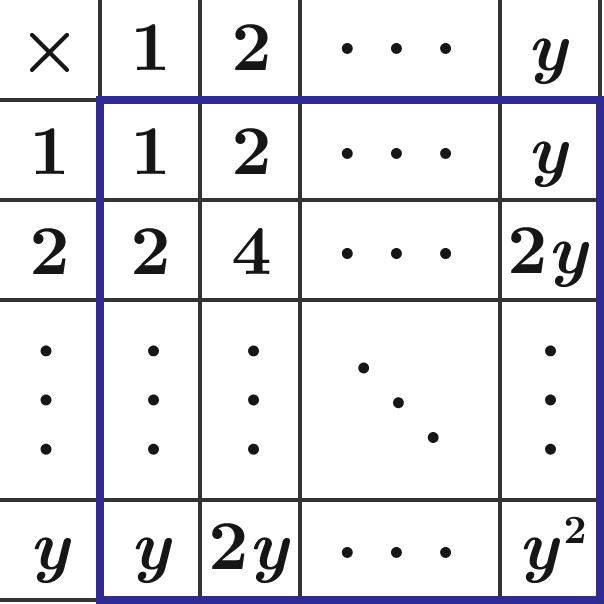Below is the graph of the equation $4x^2=y(1-y)(2-y)^2$ rotated about the $y$-axis.

Find the volume of the egg.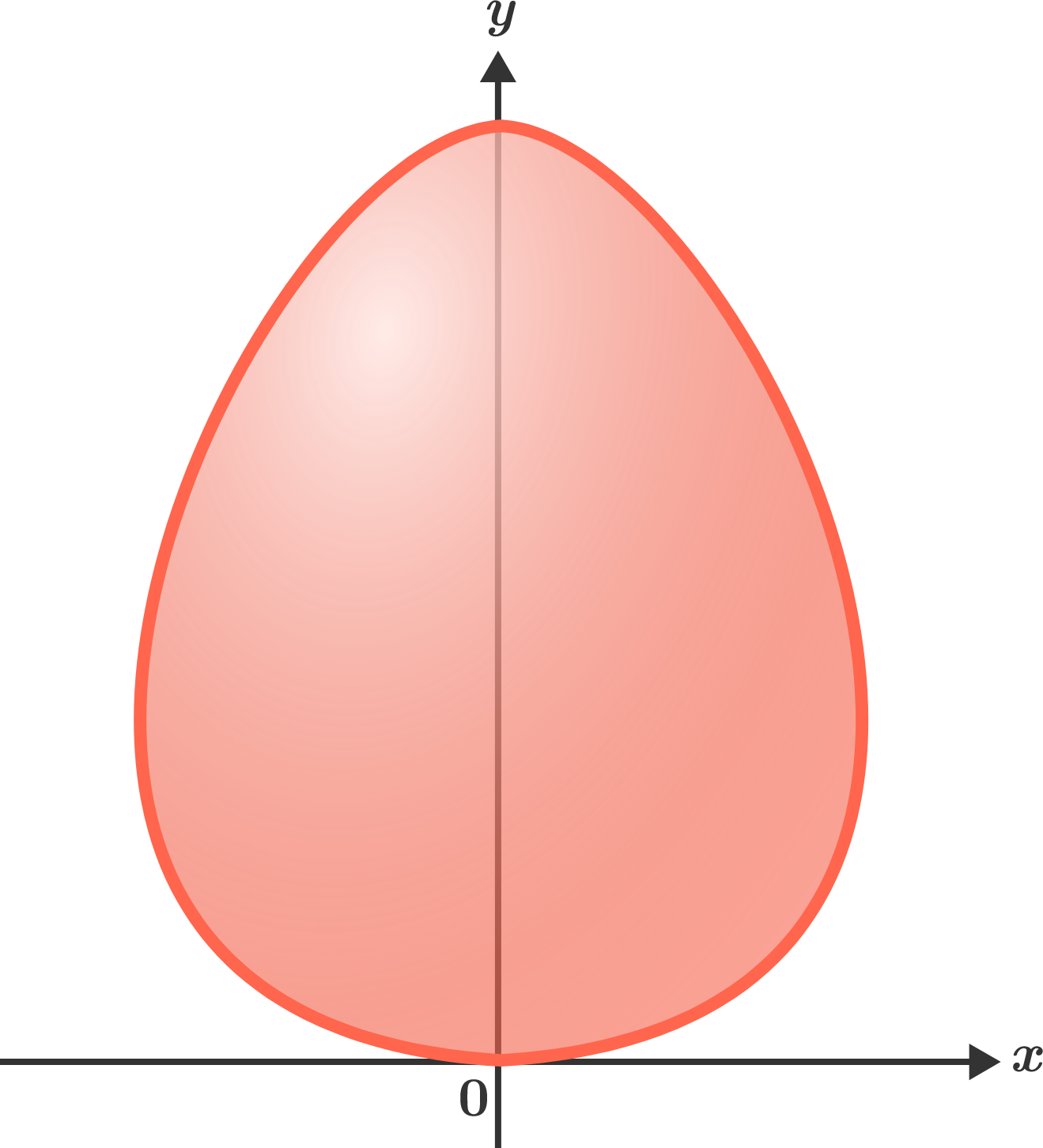×